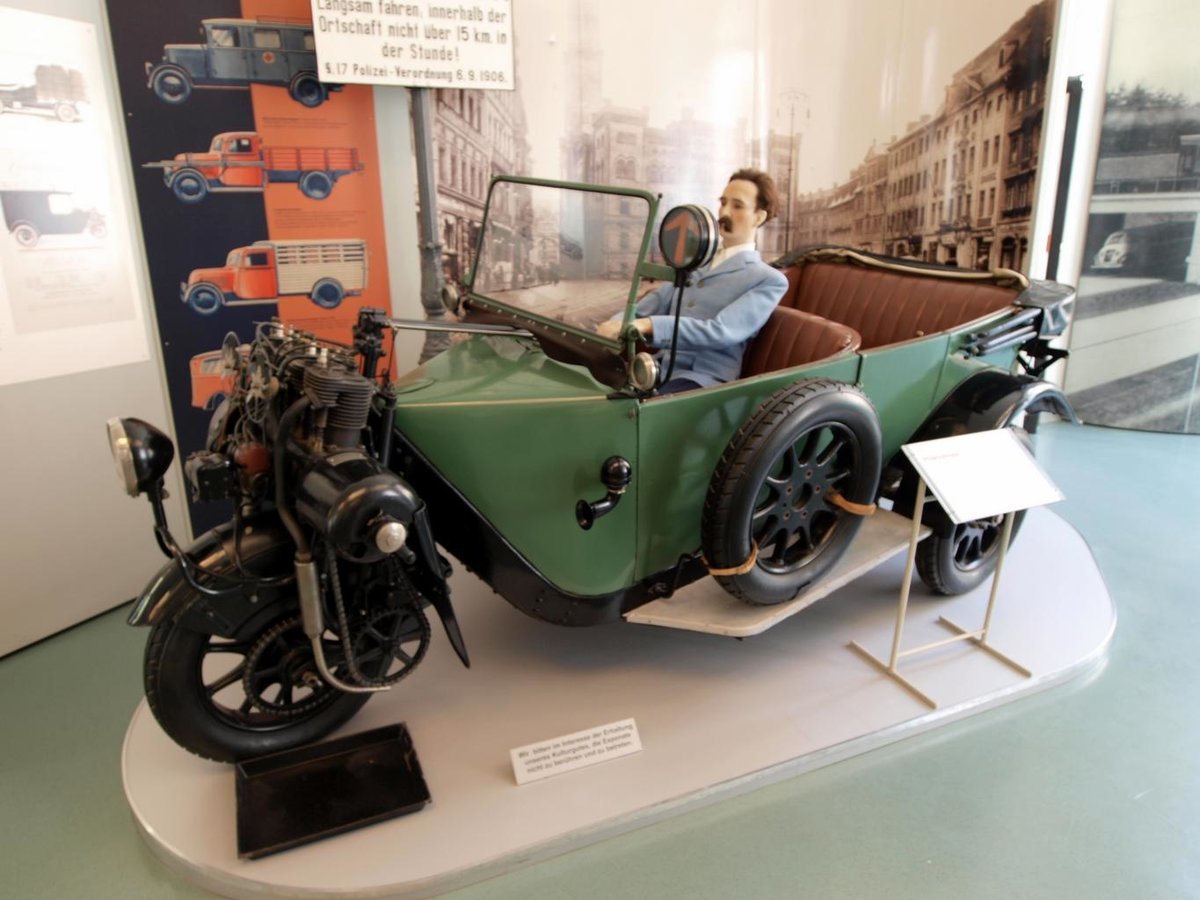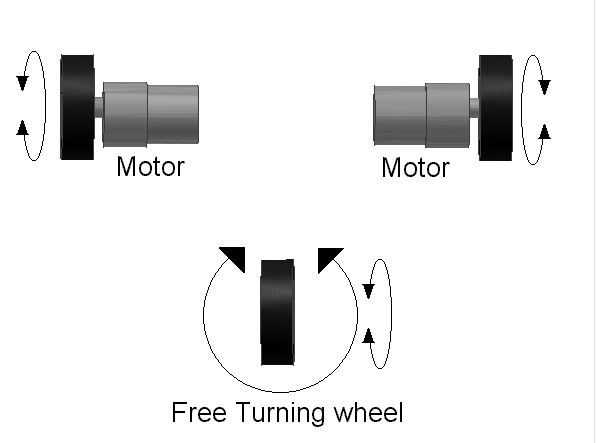# Impact of Different Sized Wheels on a Tripod-Wheeled RobotConsider a tripod-wheeled (i.e. three-wheeled) unmanned ground vehicle whose motors and wheels look like this:Two rear wheels that are connected by an axle and a free turning wheel that is in the front.

How will the vehicle move if the right rear wheel is larger than the left? Assume that the free turning wheel remains the same size, and an axle connects the left wheel to the right wheel.

The motion of a point has two types of motion, linear velocity and angular velocity. Because both wheels are connected by the same axle, the big right rear wheel and the relatively smaller left rear wheel will both rotate at the same rates. Therefore, they will have the same angular velocity.

Angular velocity (often denoted as ω) is the rate of change of the angle Ɵ. It is the number of radians (or degrees) the angle Ɵ is changing in a given amount of time.

ω = Ɵ/t

Linear velocity (denoted as v) is the rate of change of the distance traveled of the wheel. Linear velocity is related to angular velocity by the following equation, where r is the radius of the wheel.

v = rω

Therefore, the larger the wheel, the more distance it will travel given the same amount of rotation.

What does this mean for the tripod vehicle that has a right rear wheel that is larger than the left? The right rear wheel is going to cover a lot more distance on the ground in a given amount of time than the left rear wheel it is connected to. This means that, assuming the vehicle is trying to go forward, the vehicle will continuously move in a counter-clockwise direction.

Here is the diagram. Note that the big wheel has a larger radius (denoted as R) than the smaller wheel (denoted as r):

How will the vehicle move if the front turning wheel is larger than the two rear wheels? Assume the two rear wheels are equal in size and are connected by a single axle.

A larger wheel will cover a greater distance over the ground than a smaller wheel given the same amount of rotation (in either radians or degrees) per unit time. Therefore, equipping the vehicle with a larger steering wheel will enable the vehicle to move faster, holding all other wheels constant at ‘normal’.

In fact, the penny-farthing bicycles of the 1800s as well as the big wheel tricycles of the 1970s took advantage of this fact. Each had a large front wheel (and two normal rear wheels) that enabled the vehicle to move faster given a certain amount of rotation (i.e. pedaling in those cases).